## FANDOM

1,244 Pages

•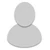## Raw gems...What do I do with them, where jewelers/workshop

April 20, 2020 by AcezAreWild

Raw gems by truckloads, but can't refine or use them, am I missing something, is there a jeweller in town or workshop I have to build/unlock..?

•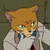## Wiki Manager introduction

March 26, 2020 by Thatawesomecat

Hello Harvest Town Wiki, I'm Thatawesomecat, your Wiki Manager here! I'll be acting as a liaison between you and Fandom Staff. For any questions or issues related to editing here, please feel free to reach out to me!

I will be around to check your Recent Changes occasionally, but if you need me, please feel free to send me a message on my talk page here. I look forward to working with you!

Thatawesomecat (talk) 20:19, March 26, 2020 (UTC)Thatawesomecat

•## Can anyone help me with Peter Allen quest that i must give gold ingkit, where can i find the gold inglot?

February 6, 2020 by Rindusari
•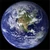## Videos?

January 2, 2020 by Ruyeex

I think this wiki needs more videos because can be a Helpful and Easy Way to do follow the action.

•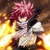## Duplicate Files

December 21, 2019 by Wildheart970

Just somewhere for me to list duplicate files before I add deletion tags. The following list includes files that are different items but share a sprite, old .jpg files that have since been replaced with .png files, and files that are of the same item but used in multiple different places. personal note: 20

• =
• =
• =
• =
• =
• =
• =
• =
• =
• =
• =
• =
• = =
• =
• =
• =
• =
• =
• =
• =
• =
• =
• =
• = =
• =
• =
• =
• =
• =
• =
• =
• =
• = = =
• =
• = =
• =
• =
• =
• =
• =
• =
• =
• =
• =
• =
• = =
• =
• =
• =
• =
• =
• =
• =
• =
• =
• =
• =
• = =
• =
• =
• =
• =
• =
• =
• =
• = =
• =
• =
• =
• =
• =
• =
• =
• =
• =
• =
• =
• =
• =
• =
• =
• =
• = =
• = =
• =
• =
• =
• = =
• =
• =
• =
• =
• =
• = =
• =
• =
• =
• =
• =
• =
• = = =
• = =
• = =
• = =
• = =
• = =
• = =
• = =
• = =
• = =
• =
• = =
• =
• =
• =
• =
• =
• =
• =
• =
• =
• =
• = =
• =
• =
• =
• =
• =
• =
• =
• =
• =
• =
• =
• =
• =
• =
• =
• =
• =
• =
• =
• =
• =
• =
• =
• =
• =
• =
• =
• =
• = =
• =
• =
• =
• =
• =
• = =
• =
• =
• =
• = =
• =
• =
• =
• =
• =
• =
• =
• =
• =
• =
• =
• = =
• =
• =
• =
• =
• =
• =
• =
• =
• =
• = =
• = =
• =
• = =
• =
• =
• =
• =
• =
• =
• = =
• =
• =
• =
• =
• =
• =
• =
• =
• = =
• =
• =
• =
• =
• =
• =
• = =
• =
• =
• = =
• =
• =
• =
• =
• =
• =
• =
• =
• =
• = =
• =
• =
• = =
• =
• =
• =
• =
• =
• =
• =
• =
• =
• =
• =
• =
• = =
• =
• =
• =
• =
• =
• =
•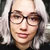December 16, 2019 by Mothkeeper

Hello! Here is a list of helpful articles and other information to help!

• Created a Sandbox - Test anything you want, whenever you want! --->
1. https://community.fandom.com/wiki/Community_Central:Sandbox
•## open the second manor beside my manor?

hi all

nice to know u all.., i newbie bout this game.

just want ask something, how to open new farm beside my manor?

thx guys

•## Wiki Wish List

December 2, 2019 by Mothkeeper

Hey folks!

I'm looking to gather your ideas for the wiki page! Our community has grown so much since the beginning and with the game still being in beta, we will be updating and growing even more. What do you think we need or can do to improve our wiki as well as our community?

•December 1, 2019 by Ruyeex

Maybe one day somebody can be the new admin to help to grow this wiki.

•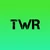## The wiki has changed a lot!

November 17, 2019 by ItzRyhere

Hi everyone!

I noticed that there are lots of changes in the Harvest Town wiki, such as a new logo, badges, new pages, and so on, since I went offline from this wiki, which is quite cool!

TheTowerOfRy

•## discord??

November 16, 2019 by Kazmierka

so there was an expired link to the discord, can we make a new one or if there is an existing one can someone share the link?

•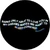## Slime monsters!!!

October 23, 2019 by Gentlekitty4

So i got this quest because i completed a part of the newest updated parchment quest

I have absolutely no idea on how to kill the slime creatures up. I've attacked them with the sword until they turn into a puddle then use a bomb, no luck. And ive tried to let them walk onto a bomb and it blow them up, but it doesnt work either.

Can anyone help me???

•## A group chat for the wiki?

October 18, 2019 by ItzRyhere

Hi there,

I am planning to create either a Facebook group or a Discord server, but do we need a group chat for this wiki? Which option is more suitable and convinient for you all? Please vote in this poll!

Thanks!!!

Update: A Discord server has been created by someone, so I am currently thinking of creating a Facebook group. Before this, I had an idea of creating both a Facebook group and Discord server as the votes for Discord were the most, and most of the news and updates comes from the Harvest Town Facebook page. What do y'all think?

•October 16, 2019 by Mothkeeper

Hello!

Our current admin is not active and does not contribute. I have filled out an adoption application and was told we needed to talk about me becoming admin and see if everyone is okay with it. In one week, I will talk to the staff member again about the adoption.

There are other people editing who may be interested in becoming admin, this should be something we discuss and vote to elect a new admin.

The poll is here: Poll

UPDATE: I was notified that poll votes do not count for verifying with everyone here that you are okay with me becoming admin (polls can be manipulated, like if someone were to have multiple users, etc.).

Could I have you guys comment here with your thoughts on me becoming admin? Even a yay or nay or something would be n…

•## Help with editting the recipes

October 5, 2019 by ItzRyhere

Hi there! I need help with editting all of the recipes in Cuisine.

The templete for the recipes is gone (idk how) and all of the recipes have blank pages.

I have created a new templete, which is RecipeBox, and I need help with changing all of the récipe templete into RecipeBox templetes. Thanks a lot!!!

Example

{{RecipeBox

••